## Sinc Function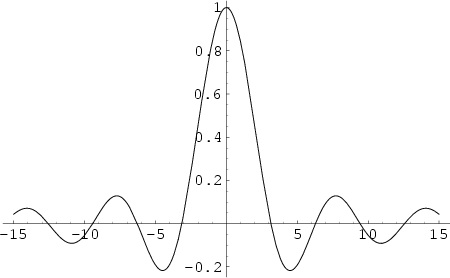A function also called the Sampling Function and defined by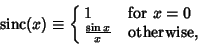(1)

where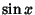is the Sine function. Let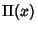be the Rectangle Function, then the Fourier Transform ofis the sinc function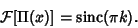(2)

The sinc function therefore frequently arises in physical applications such as Fourier transform spectroscopyas the so-called Instrument Function, which gives the instrumental response to a Delta Function input. Removing the instrument functions from the final spectrum requires use of some sort of Deconvolution algorithm.

The sinc function can be written as a complex Integral by noting that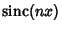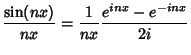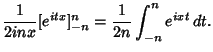(3)

The sinc function can also be written as the Infinite Product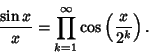(4)

Definite integrals involving the sinc function include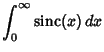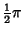(5)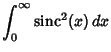(6)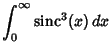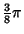(7)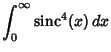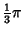(8)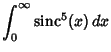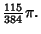(9)

These are all special cases of the amazing general result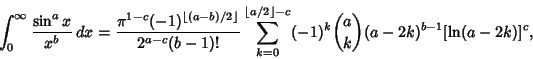(10)

whereand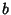are Positive integers such that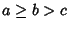,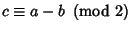,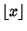is the Floor Function, and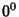is taken to be equal to 1 (Kogan). This spectacular formula simplifies in the special case whenis a Positive Even integer to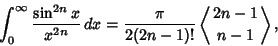(11)

where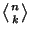is an Eulerian Number (Kogan). The solution of the integral can also be written in terms of the Recurrence Relation for the coefficients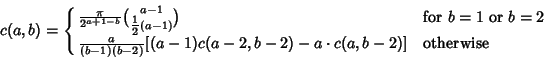(12)

(Zimmerman).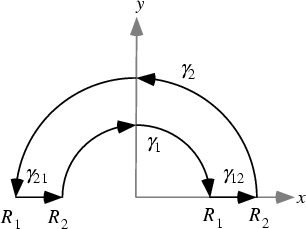The half-infinite integral of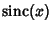can be derived using Contour Integration. In the above figure, consider the path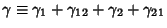. Now write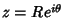. On an arc,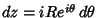and on the x-Axis,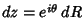. Write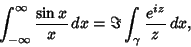(13)

where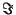denotes the Imaginary Point. Now define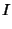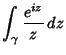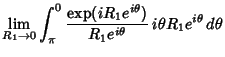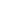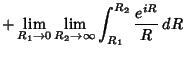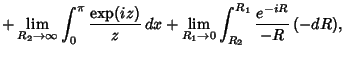(14)

where the second and fourth terms use the identities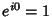and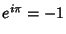. Simplifying,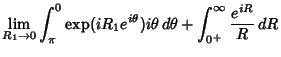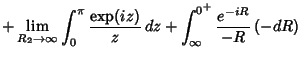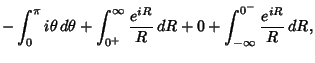(15)

where the third term vanishes by Jordan's Lemma. Performing the integration of the first term and combining the others yield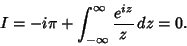(16)

Rearranging gives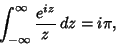(17)

so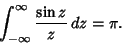(18)

The same result is arrived at using the method of Residues by noting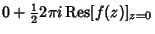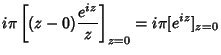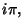(19)

so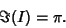(20)

Since the integrand is symmetric, we therefore have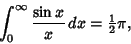(21)

giving the Sine Integral evaluated at 0 as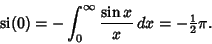(22)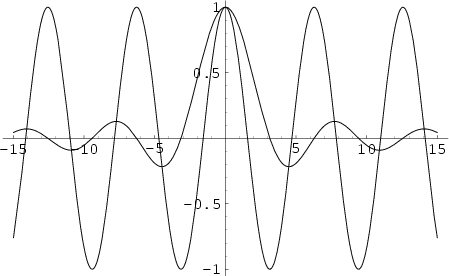An interesting property ofis that the set of Local Extrema ofcorresponds to its intersections with the Cosine function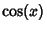, as illustrated above.

See also Fourier Transform, Fourier Transform--Rectangle Function, Instrument Function, Jinc Function, Sine, Sine Integral

References

Kogan, S. A Note on Definite Integrals Involving Trigonometric Functions.'' http://www.mathsoft.com/asolve/constant/pi/sin/sin.html.

Morrison, K. E. Cosine Products, Fourier Transforms, and Random Sums.'' Amer. Math. Monthly 102, 716-724, 1995.